# How to Auto-Number a Column in Excel?

Have you ever tried to create a list of numbers in Excel? It is a very simple process, and there are many ways to do it. Generally, this kind of list is generated to assign the serial numbers to the list and also count the number of rows we used.

Read this to learn the different ways to auto-number a column in Excel. In this tutorial, we will look at three different methods for creating a series: the row function, the offset function, and simply using the auto-fill handle.

## Auto-Number a Column Using the Row function

Here we will Enter the formula in the first cell and drag down to get a list of numbers. Let us see a simple process to auto-number a column using the row function in Excel.

Step 1

Consider creating a new Excel sheet and entering the formula =ROW() in the first cell and pressing enter, as shown in the image below.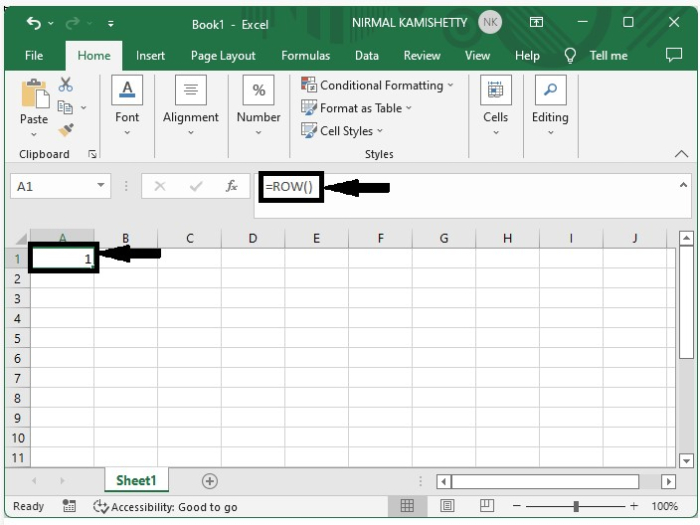Step 2

As we have the first result, we can get a number of results just by dragging down from the corner of the first cell, and our final output will be similar to the data represented in the below image.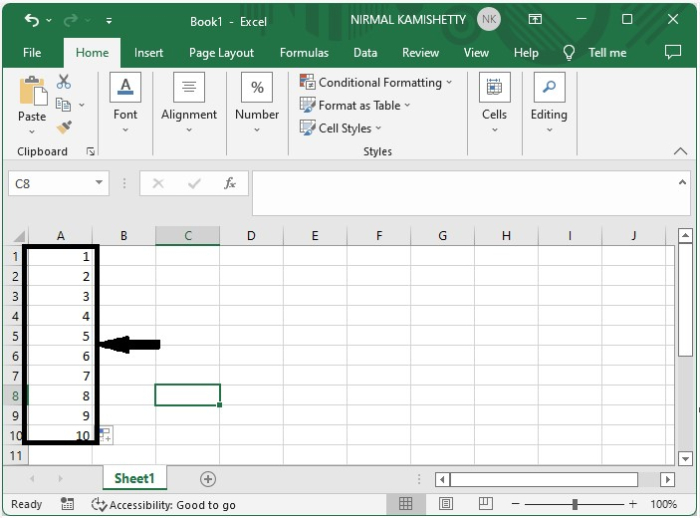## Auto-Number a Column Using the Offset Function

Enter the formula in the first cell and drag down to get a list of numbers. Let us see a simple process to auto-number a column using the offset function.

Step 1

Let us consider an empty excel sheet and enter the formula as =OFFSET(A2,-1,0)+1 in the first cell and click on "Enter" as shown in the below image. Here in the formula, A2, is the current address, and to use this formula, we have to make sure that the above cell is always empty, in our case, the cell A1.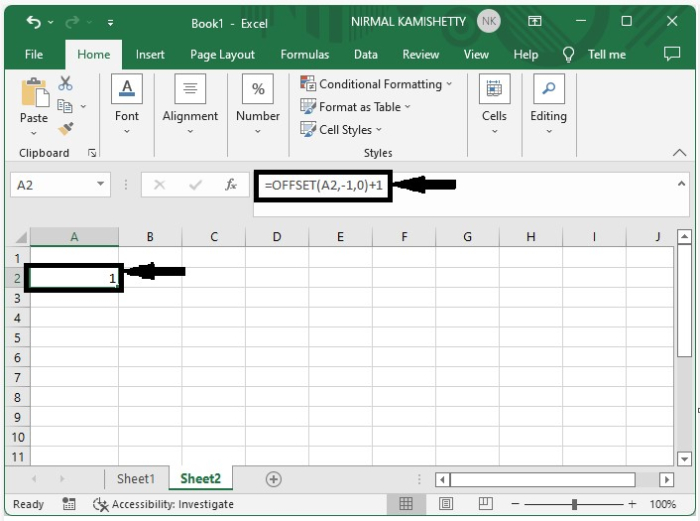Step 2

As we have the first result, we can get a number of results just by dragging down from the corner of the first cell, and our final output will be similar to the data represented in the below image.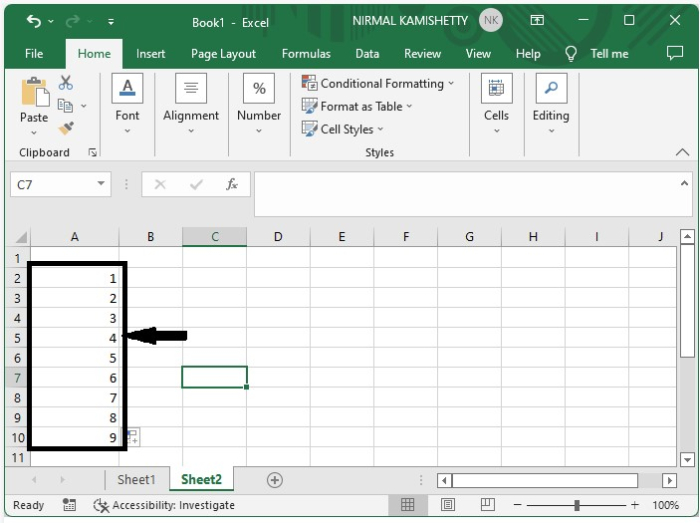## Auto-Number a Column Using the Auto-Fill Handle

Here we will enter one in the first cell, then drag down to get the list of numbers. Let us see a simple process to auto-number a column using the auto-fill handle.

It is the simplest process among the three. The only thing is that we need to make sure that the fill handle is selected for the fill series.

Here, in the new sheet, enter "1" in the first cell and drag down until you have as many results as you want to print in the sheet; the output will be similar to the below image.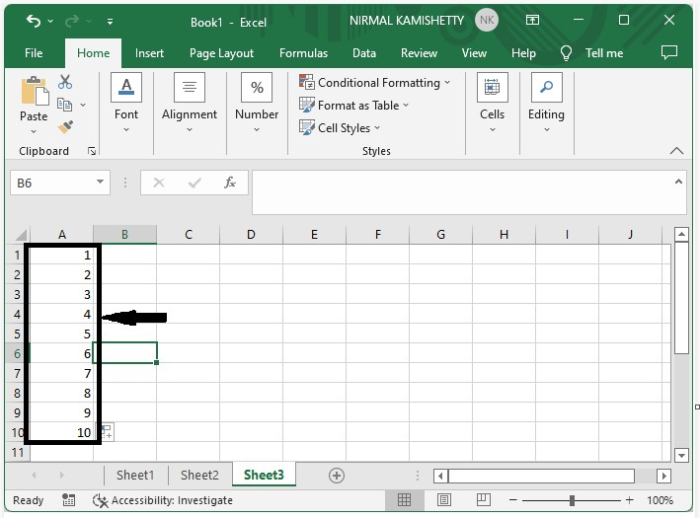## Conclusion

In this tutorial, we used some simple examples to demonstrate how you can auto-number a column in Excel.

Updated on: 11-Jan-2023

162 Views